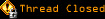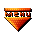# Topic awaiting preservation: How can I pass variable value from vbscript to JavaScript? (Page 1 of 1)Hiroki
Paranoid (IV) Inmate

From: NZ
Insane since: Dec 2002posted 11-06-2003 22:28

Hi, guys. How are you?
I am trying to pass vbscript variable value to Javascript.
Some reason, doesn't work.
I have code in body like:

code:
```<%Do Until rs.EOF or recct >=maxrecs%>
<tr>
<% For each x in rs.Fields %>

<%If x.name="code" Then%>
<td><img src="../assets/Pendants/<%'Response.Write(x.value)%>"></td>
<%End If%>
<%If x.name <> "catagory" Then%>
<td><% If x.name = "price" Then  Response.Write("\$" & x.value) Else Response.Write(x.value) End IF %></td>

<%END IF%>
<%Next%>
<%rs.MoveNext
[b] recct = recct + 1[/b]
%>
<td><input type="text" name="quant" value="0"></td>
</tr>
<%Loop%>```

code:
```<script type="text/javascript">
function calculate(){
var quants = document.getElementsByName("quant");
var total = 0;
var cost = 0;
for(i=1; i<=[b]recct[/b]; i++){
var quant = parseInt(quants.item(i-1).value);
cost = quant;
total = total + cost;
}
document.theForm.total.value = total;
}
</script>```

In JavaScript, I want to run loop recct times which is coming from VBscript code above.
I have to declare variable in JavaScript. But I cannot figure out how.
Do you know what I mean?

Hiroki Kozai

bitdamaged

From: 100101010011 <-- right about here
Insane since: Mar 2000posted 11-07-2003 01:29

I'm not an asp guy but you should be able to just Response.Write the value of recct in the Javascript..:[ Never resist a perfect moment ]:.

nipgnik
Neurotic (0) Inmate

From: Maine, USA
Insane since: Nov 2003posted 11-08-2003 04:26

<%
response.write "<script language='JavaScript'>"
response.write "<!--"
response.write "var recct = " & recct
response.write "//--></script>"
%>

this should work.

Bipolar (III) Inmate

From: Israel
Insane since: Nov 2001posted 11-09-2003 11:06

Hi,
I don't see why you need the "recct" parameter, you can use the quants.length instead.

<script type="text/javascript">
function calculate(){
var quants = document.getElementsByName("quant");
var total = 0;
var cost = 0;
for(i=0; i<=quants.length; i++){
var quant = parseInt(quants.item(i).value);
cost = quant;
total = total + cost;
}
document.theForm.total.value = total;
}
</script>